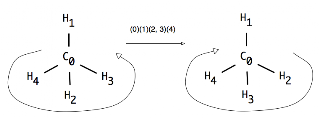Skip to main content

### External Symmetry Numbers and Graph Automorphism Groups

So, there was a question on BioStar about calculating the 'external symmetry number' of a molecule - something I hadn't heard of, but turns out to be something like the subgroup of rotations and reflections of the automorphism group of a graph. Since I have some code to calculate the automorphism group, I naïvely thought it would be simple...

The questioner - Nick Vandewiele - kindly provided some test cases, which ended up as this code. Although many of these tests now pass, they only do so because I commented out the hydrogen adding! :)

On the one hand, there are some recent improvements that try to handle vertex and edge 'colors' - in other words, element symbols and bond orders. For example, consider the improbable molecule C1OCO1 :
These are the three permutations that leave the carbons and the oxygens in the same positions; when you include the identity, that makes 4. Cyclobutane (without hydrogens!) has a symmetry group of order 8. Similarly, cyclobutadiene now gives 4 instead of 8.

So what goes wrong when there are hydrogens? Well, it's a deeper problem than just hydrogens, but it starts there. Consider methane : it has an external symmetry number of 12, but my code gives 24 - why? Well the main answer is 'inversion', look:The permutation (0)(1)(2, 3)(4) just swaps hydrogens 2 and 3. This effectively changes the chirality of the molecule ... sortof. It's not actually chiral, but its a reasonable description of the transformation. Apparently, this does happen (another thing I didn't know; there are lots more :) according to this document, but quite slowly compared to rotations - "slower than 1 cycle s-1".

This kind of pseudo-chirality will happen at any tetrahedral center. Or at any atom with 4 neighbours, I think - like XeF4, which is square planar. As an example, take this spira-fused ring system:with a transform that swaps 7 and 9 but not the pairs (0, 5)(1, 4)(2, 3). Effectively this changes the parity at carbon 6. Somehow I doubt that this kind of 'movement' actually occurs in solution, but I could well be wrong. In any case, it seems likely that the external symmetry number is 2, and not 4.

In summary, it is probably not possible to calculate the external symmetry number correctly without 3D coordinates, or symmetry axes, or point groups. I have a feeling that the positional info could be recorded as a 3D combinatorial map which would give explicit orientations for atoms with four neighbours.

### How many isomers of C4H11N are there?

One of the most popular queries that lands people at this blog is about the isomers of C4H11N - which I suspect may be some kind of organic chemistry question on student homework. In any case, this post will describe how to find all members of a small space like this by hand rather than using software.

Firstly, lets connect all the hydrogens to the heavy atoms (C and N, in this case). For example:

Now eleven hydrogens can be distributed among these five heavy atoms in various ways. In fact this is the problem of partitioning a number into a list of other numbers which I've talked about before. These partitions and (possible) fragment lists are shown here:

One thing to notice is that all partitions have to have 5 parts - even if one of those parts is 0. That's not strictly a partition anymore, but never mind. The other important point is that some of the partitions lead to multiple fragment lists - [3, 3, 2, 2, 1] could have a CH+NH2 or an NH+CH2.

The final step is to connect u…

### The Gale-Ryser Theorem

This is a small aside. While reading a paper by Grüner, Laue, and Meringer on generation by homomorphism they mentioned the Gale-Ryser (GR) theorem. As it turns out, this is a nice small theorem closely related to the better known Erdős-Gallai (EG).

So, GR says that given two partitions of an integer (p and q) there exists a (0, 1) matrixA iff p*dominatesq such that the row sum vector r(A) = p and the column sum vector c(A) = q.

As with most mathematics, that's quite terse and full of terminology like 'dominates' : but it's relatively simple. Here is an example:

The partitions p and q are at the top left, they both sum to 10. Next, p is transposed to get p* = [5, 4, 1] and this is compared to q at the bottom left. Since the sum at each point in the sequence is greater (or equal) for p* than q, the former dominates. One possible matrix is at the top left with the row sum vector to the right, and the column sum vector below.

Finally, the matrix can be interpreted as a bi…

### Havel-Hakimi Algorithm for Generating Graphs from Degree Sequences

A degree sequence is an ordered list of degrees for the vertices of a graph. For example, here are some graphs and their degree sequences:

Clearly, each graph has only one degree sequence, but the reverse is not true - one degree sequence can correspond to many graphs. Finally, an ordered sequence of numbers (d1 >= d2 >= ... >= dn > 0) may not be the degree sequence of a graph - in other words, it is not graphical.

The Havel-Hakimi (HH) theorem gives us a way to test a degree sequence to see if it is graphical or not. As a side-effect, a graph is produced that realises the sequence. Note that it only produces one graph, not all of them. It proceeds by attaching the first vertex of highest degree to the next set of high-degree vertices. If there are none left to attach to, it has either used up all the sequence to produce a graph, or the sequence was not graphical.

The image above shows the HH algorithm at work on the sequence [3, 3, 2, 2, 1, 1]. Unfortunately, this produce…